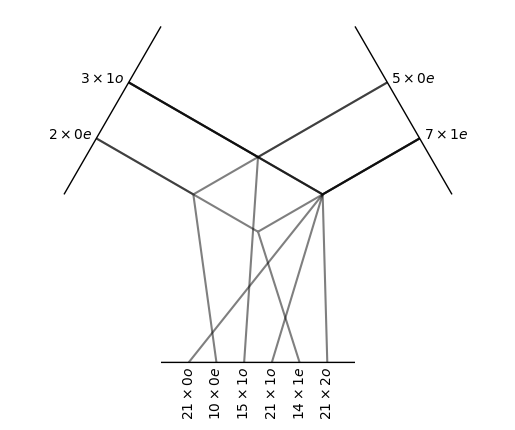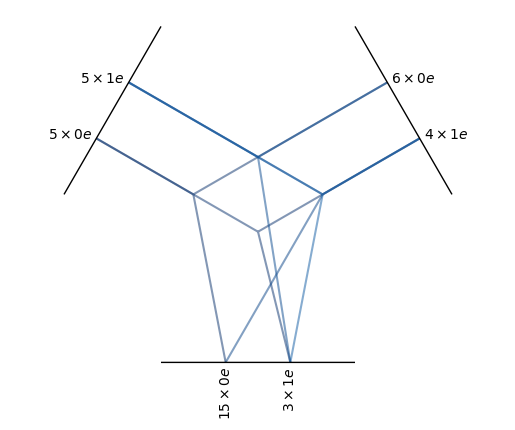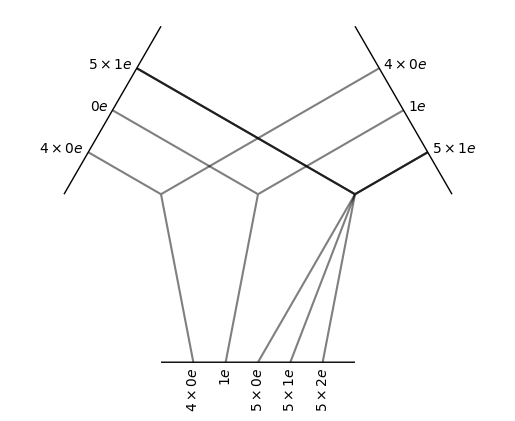# Tensor Product¶

Two characteristics of all tensor products (denoted $$\otimes$$) are:

1. The tensor product is bilinear: $$(\alpha x_1 + x_2) \otimes y = \alpha x_1 \otimes y + x_2 \otimes y$$ and $$x \otimes (\alpha y_1 + y_2) = \alpha x \otimes y_1 + x \otimes y_2$$

2. The tensor product is equivariant: $$(D x) \otimes (D y) = D (x \otimes y)$$ (sorry for the very loose notation)

The class TensorProduct implements all tensor products of finite direct sums of irreducible representations (Irreps).

All the classes here inherit from the class TensorProduct. Each class implements a special case of tensor product.

o3.FullTensorProduct('2x0e + 3x1o', '5x0e + 7x1e').visualize()

(<Figure size 432x288 with 1 Axes>, <AxesSubplot:>)The full tensor product is the “natural” one. Every possible output is created and the outputs are not mixed with each other. Note how the multiplicities of the outputs are the product of the multiplicities of the respective inputs.

o3.FullyConnectedTensorProduct('5x0e + 5x1e', '6x0e + 4x1e', '15x0e + 3x1e').visualize()

(<Figure size 432x288 with 1 Axes>, <AxesSubplot:>)In a fully connected tensor product, all possible paths are created. The outputs are mixed together with learnable parameters. The red color indicates that the path is learned.

o3.ElementwiseTensorProduct('5x0e + 5x1e', '4x0e + 6x1e').visualize()

(<Figure size 432x288 with 1 Axes>, <AxesSubplot:>)In the elementwise tensor product, the irreps are multiplied one by one. Note how the inputs have been split and how the multiplicities of the outputs match with the multiplicities of the input.

class e3nn.o3.TensorProduct(irreps_in1: e3nn.o3._irreps.Irreps, irreps_in2: e3nn.o3._irreps.Irreps, irreps_out: e3nn.o3._irreps.Irreps, instructions: List[tuple], in1_var: Optional[Union[List[float], torch.Tensor]] = None, in2_var: Optional[Union[List[float], torch.Tensor]] = None, out_var: Optional[Union[List[float], torch.Tensor]] = None, normalization: str = 'component', internal_weights: Optional[bool] = None, shared_weights: Optional[bool] = None, _specialized_code: Optional[bool] = None, _optimize_einsums: Optional[bool] = None)

Bases: e3nn.util.codegen._mixin.CodeGenMixin, torch.nn.modules.module.Module

Tensor product with parametrized paths.

Parameters
• irreps_in1 (Irreps) – Irreps for the first input.

• irreps_in2 (Irreps) – Irreps for the second input.

• irreps_out (Irreps) – Irreps for the output.

• instructions (list of tuple) –

List of instructions (i_1, i_2, i_out, mode, train[, path_weight]).

Each instruction puts in1[i_1] $$\otimes$$ in2[i_2] into out[i_out].

• in1_var (list of float, Tensor, or None) – Variance for each irrep in irreps_in1. If None, all default to 1.0.

• in2_var (list of float, Tensor, or None) – Variance for each irrep in irreps_in2. If None, all default to 1.0.

• out_var (list of float, Tensor, or None) – Variance for each irrep in irreps_out. If None, all default to 1.0.

• normalization ({'component', 'norm'}) –

The assumed normalization of representations. If it is set to “norm”:

$\| x \| = \| y \| = 1 \Longrightarrow \| x \otimes y \| = 1$
• internal_weights (bool) – does the instance of the class contains the parameters

• shared_weights (bool) –

are the parameters shared among the inputs extra dimensions

where here $$i$$ denotes a batch-like index

Examples

Create a module that computes elementwise the cross-product of 16 vectors with 16 vectors $$z_u = x_u \wedge y_u$$

>>> module = TensorProduct(
...     "16x1o", "16x1o", "16x1e",
...     [
...         (0, 0, 0, "uuu", False)
...     ]
... )


Now mix all 16 vectors with all 16 vectors to makes 16 pseudo-vectors $$z_w = \sum_{u,v} w_{uvw} x_u \wedge y_v$$

>>> module = TensorProduct(
...     [(16, (1, -1))],
...     [(16, (1, -1))],
...     [(16, (1,  1))],
...     [
...         (0, 0, 0, "uvw", True)
...     ]
... )


With custom input variance and custom path weights:

>>> module = TensorProduct(
...     "8x0o + 8x1o",
...     "16x1o",
...     "16x1e",
...     [
...         (0, 0, 0, "uvw", True, 3),
...         (1, 0, 0, "uvw", True, 1),
...     ],
...     in2_var=[1/16]
... )


Example of a dot product:

>>> irreps = o3.Irreps("3x0e + 4x0o + 1e + 2o + 3o")
>>> module = TensorProduct(irreps, irreps, "0e", [
...     (i, i, 0, 'uuw', False)
...     for i, (mul, ir) in enumerate(irreps)
... ])


Implement $$z_u = x_u \otimes (\sum_v w_{uv} y_v)$$

>>> module = TensorProduct(
...     "8x0o + 7x1o + 3x2e",
...     "10x0e + 10x1e + 10x2e",
...     "8x0o + 7x1o + 3x2e",
...     [
...         # paths for the l=0:
...         (0, 0, 0, "uvu", True),  # 0x0->0
...         # paths for the l=1:
...         (1, 0, 1, "uvu", True),  # 1x0->1
...         (1, 1, 1, "uvu", True),  # 1x1->1
...         (1, 2, 1, "uvu", True),  # 1x2->1
...         # paths for the l=2:
...         (2, 0, 2, "uvu", True),  # 2x0->2
...         (2, 1, 2, "uvu", True),  # 2x1->2
...         (2, 2, 2, "uvu", True),  # 2x2->2
...     ]
... )


Tensor Product using the xavier uniform initialization:

>>> irreps_1 = o3.Irreps("5x0e + 10x1o + 1x2e")
>>> irreps_2 = o3.Irreps("5x0e + 10x1o + 1x2e")
>>> irreps_out = o3.Irreps("5x0e + 10x1o + 1x2e")
>>> # create a Fully Connected Tensor Product
>>> module = o3.TensorProduct(
...     irreps_1,
...     irreps_2,
...     irreps_out,
...     [
...         (i_1, i_2, i_out, "uvw", True, mul_1 * mul_2)
...         for i_1, (mul_1, ir_1) in enumerate(irreps_1)
...         for i_2, (mul_2, ir_2) in enumerate(irreps_2)
...         for i_out, (mul_out, ir_out) in enumerate(irreps_out)
...         if ir_out in ir_1 * ir_2
...     ]
... )
>>> with torch.no_grad():
...     for weight in module.weight_views():
...         mul_1, mul_2, mul_out = weight.shape
...         # formula from torch.nn.init.xavier_uniform_
...         a = (6 / (mul_1 * mul_2 + mul_out))**0.5
...         new_weight = torch.empty_like(weight)
...         new_weight.uniform_(-a, a)
...         weight[:] = new_weight
tensor(...)
>>> n = 1_000
>>> vars = module(irreps_1.randn(n, -1), irreps_2.randn(n, -1)).var(0)
>>> assert vars.min() > 1 / 3
>>> assert vars.max() < 3


Methods:

 forward(x, y[, weight]) Evaluate $$w x \otimes y$$. right(y[, weight]) Partially evaluate $$w x \otimes y$$. visualize([weight, plot_weight, ax]) Visualize the connectivity of this TensorProduct weight_view_for_instruction(instruction[, …]) View of weights corresponding to instruction. weight_views([weight, yield_instruction]) Iterator over weight views for each weighted instruction.
forward(x, y, weight: Optional[torch.Tensor] = None)

Evaluate $$w x \otimes y$$.

Parameters
• x (torch.Tensor) – tensor of shape (..., irreps_in1.dim)

• y (torch.Tensor) – tensor of shape (..., irreps_in2.dim)

• weight (torch.Tensor or list of torch.Tensor, optional) – required if internal_weights is False tensor of shape (self.weight_numel,) if shared_weights is True tensor of shape (..., self.weight_numel) if shared_weights is False or list of tensors of shapes weight_shape / (...) + weight_shape. Use self.instructions to know what are the weights used for.

Returns

tensor of shape (..., irreps_out.dim)

Return type

torch.Tensor

right(y, weight: Optional[torch.Tensor] = None)

Partially evaluate $$w x \otimes y$$.

It returns an operator in the form of a tensor that can act on an arbitrary $$x$$.

For example, if the tensor product above is expressed as

$w_{ijk} x_i y_j \rightarrow z_k$

then the right method returns a tensor $$b_{ik}$$ such that

$w_{ijk} y_j \rightarrow b_{ik} x_i b_{ik} \rightarrow z_k$

The result of this method can be applied with a tensor contraction:

torch.einsum("...ik,...i->...k", right, input)

Parameters
• y (torch.Tensor) – tensor of shape (..., irreps_in2.dim)

• weight (torch.Tensor or list of torch.Tensor, optional) – required if internal_weights is False tensor of shape (self.weight_numel,) if shared_weights is True tensor of shape (..., self.weight_numel) if shared_weights is False or list of tensors of shapes weight_shape / (...) + weight_shape. Use self.instructions to know what are the weights used for.

Returns

tensor of shape (..., irreps_in1.dim, irreps_out.dim)

Return type

torch.Tensor

visualize(weight: Optional[torch.Tensor] = None, plot_weight: bool = True, ax=None)

Visualize the connectivity of this TensorProduct

Parameters
• weight (torch.Tensor, optional) – like weight argument to forward()

• plot_weight (bool, default True) – Whether to color paths by the sum of their weights.

• ax (matplotlib.Axes, default None) – The axes to plot on. If None, a new figure will be created.

Returns

The figure and axes on which the plot was drawn.

Return type

(fig, ax)

weight_view_for_instruction(instruction: int, weight: Optional[torch.Tensor] = None)torch.Tensor

View of weights corresponding to instruction.

Parameters
• instruction (int) – The index of the instruction to get a view on the weights for. self.instructions[instruction].has_weight must be True.

• weight (torch.Tensor, optional) – like weight argument to forward()

Returns

A view on weight or this object’s internal weights for the weights corresponding to the instruction th instruction.

Return type

torch.Tensor

weight_views(weight: Optional[torch.Tensor] = None, yield_instruction: bool = False)

Iterator over weight views for each weighted instruction.

Parameters
• weight (torch.Tensor, optional) – like weight argument to forward()

• yield_instruction (bool, default False) – Whether to also yield the corresponding instruction.

Yields
• If yield_instruction is True, yields (instruction_index, instruction, weight_view).

• Otherwise, yields weight_view.

class e3nn.o3.FullyConnectedTensorProduct(irreps_in1, irreps_in2, irreps_out, **kwargs)

Bases: e3nn.o3._tensor_product._tensor_product.TensorProduct

Fully-connected weighted tensor product

All the possible path allowed by $$|l_1 - l_2| \leq l_{out} \leq l_1 + l_2$$ are made. The output is a sum on different paths:

$z_w = \sum_{u,v} w_{uvw} x_u \otimes y_v + \cdots \text{other paths}$

where $$u,v,w$$ are the indices of the multiplicities.

Parameters
class e3nn.o3.FullTensorProduct(irreps_in1, irreps_in2, filter_ir_out=None, **kwargs)

Bases: e3nn.o3._tensor_product._tensor_product.TensorProduct

Full tensor product between two irreps.

$z_{uv} = x_u \otimes y_v$

where $$u$$ and $$v$$ run over the irreps. Note that there are no weights.

Parameters
class e3nn.o3.ElementwiseTensorProduct(irreps_in1, irreps_in2, filter_ir_out=None, **kwargs)

Bases: e3nn.o3._tensor_product._tensor_product.TensorProduct

Elementwise connected tensor product.

$z_u = x_u \otimes y_u$

where $$u$$ runs over the irreps. Note that there are no weights.

Parameters